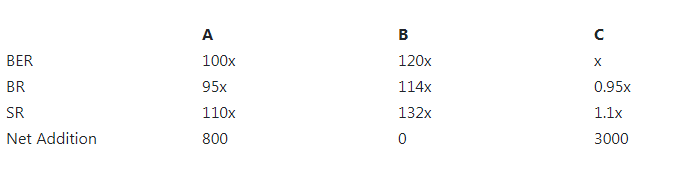# The base exchange rate of a currency | CAT DILR Questions | Logical Reasoning | Puzzle

## CAT 2018 – Slot 2 - Logical Reasoning - The base exchange rate of a currency

##### The base exchange rate of a currency X with respect to a currency Y is the number of units of currency Y which is equivalent in value to one unit of currency X. Currency exchange outlets buy currency at buying exchange rates that are lower than base exchange rates, and sell currency at selling exchange rates that are higher than base exchange rates. A currency exchange outlet uses the local currency L to buy and sell three international currencies A, B, and C, but does not exchange one international currency directly with another. The base exchange rates of A, B and C with respect to L are in the ratio 100:120:1. The buying exchange rates of each of A, B, and C with respect to L are 5% below the corresponding base exchange rates, and their selling exchange rates are 10% above their corresponding base exchange rates. The following facts are known about the outlet on a particular day: 1. The amount of L used by the outlet to buy C equals the amount of L it received by selling C. 2. The amounts of L used by the outlet to buy A and B are in the ratio 5:3. 3. The amounts of L the outlet received from the sales of A and B are in the ratio 5:9. 4. The outlet received 88000 units of L by selling A during the day. 5. The outlet started the day with some amount of L, 2500 units of A, 4800 units of B, and 48000 units of C. 6. The outlet ended the day with some amount of L, 3300 units of A, 4800 units of B, and 51000 units of C.

Q. 1: How many units of currency A did the outlet buy on that day?

##### Q. 2: How many units of currency C did the outlet sell on that day?1. 190002. 30003. 60004. 22000

Q. 3: What was the base exchange rate of currency B with respect to currency L on that day?

Q. 4: What was the buying exchange rate of currency C with respect to currency L on that day?
1. 0.95
2. 1.10
3. 1.90
4. 2.20

The base exchange rates (BER) of currencies A, B and C with respect to L is in the ratio 100 : 120 : 1. Also The buying exchange rates (BR) of each of A, B, and C with respect to L will be in the ratio  = 95 : 114: 0.95 and corresponding selling rates (SR) will be 110, 132 and 1.1 respectively as it is 10% more than base exchange rates.
The given information can be tabulated as follows:From point 4) The outlet received 88,000 units of L by selling A.
From point 2) & 3)  the ratio of amounts of L used to by A and B are in the ratio 5 : 3 and from the sales of A and B are in the ratio 5 : 9.
Let the base exchange rates for A, B and C 100x,120x and x .
Units sold of A = 88,000/110x = 800/x.
As the net addition is 800, the units of A bought is 800 + 800/x
Amount of L used in buying 800 + 800/x units is (800 +800/x)×0.95 × 100x = 76000(x+1) .
Now As the amount used to buy A and B are in the ratio 5 : 3,
The amount used to buy B is 76000(x+1) /5 X 3 = 45,600(x+1)
Number of units of B bought = 45,600(x+1)/114x = 400(x+1)/x
As the net addition of B is zero, number of units of B sold = 400(x+1)/x
The amount received form selling A = 88,000
As given 88,000 : 52800(x+1) = 5 : 9
880/528(x+1) = 5/9
(x+1) = 880*9/558*5 = 3
x = 2
1. The units of A bought is 800 + 800/x = 800+ 800/2 = 1200

2. As the net addition in the number of units of C is 3,000 and the buying and selling rates are in the ratio 0.95 and 1.1, assuming x units are sold 0.95 (x + 3000) = 1.1 (x) 0.15x = 2850 X= 19000

3. The base exchange rate of currency B with respect to L is 120x = 120*2 = 240

4. The buying exchange rate of currency C with respect to L on that day was 0.95x = 1.9

### Past Year Question Paper & SolutionsCounselling Session
By IIM Mentor

### Free Material Area